This book is archived and will be removed July 6, 2022. Please use the updated version.

Nuclear Physics

# 74 Nuclear Binding Energy

### Learning Objectives

By the end of this section, you will be able to:

• Calculate the mass defect and binding energy for a wide range of nuclei
• Use a graph of binding energy per nucleon (BEN) versus mass number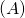graph to assess the relative stability of a nucleus
• Compare the binding energy of a nucleon in a nucleus to the ionization energy of an electron in an atom

The forces that bind nucleons together in an atomic nucleus are much greater than those that bind an electron to an atom through electrostatic attraction. This is evident by the relative sizes of the atomic nucleus and the atom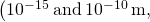respectively). The energy required to pry a nucleon from the nucleus is therefore much larger than that required to remove (or ionize) an electron in an atom. In general, all nuclear changes involve large amounts of energy per particle undergoing the reaction. This has numerous practical applications.

### Mass Defect

According to nuclear particle experiments, the total mass of a nucleus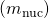is less than the sum of the masses of its constituent nucleons (protons and neutrons). The mass difference, or mass defect, is given by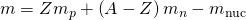where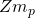is the total mass of the protons,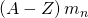is the total mass of the neutrons, and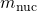is the mass of the nucleus. According to Einstein’s special theory of relativity, mass is a measure of the total energy of a system (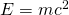). Thus, the total energy of a nucleus is less than the sum of the energies of its constituent nucleons. The formation of a nucleus from a system of isolated protons and neutrons is therefore an exothermic reaction—meaning that it releases energy. The energy emitted, or radiated, in this process is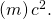Now imagine this process occurs in reverse. Instead of forming a nucleus, energy is put into the system to break apart the nucleus ((Figure)). The amount of energy required is called the total binding energy (BE),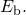Binding Energy

The binding energy is equal to the amount of energy released in forming the nucleus, and is therefore given by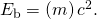Experimental results indicate that the binding energy for a nucleus with mass number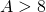is roughly proportional to the total number of nucleons in the nucleus, A. The binding energy of a magnesium nucleus (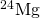), for example, is approximately two times greater than for the carbon nucleus (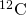).

The binding energy is the energy required to break a nucleus into its constituent protons and neutrons. A system of separated nucleons has a greater mass than a system of bound nucleons.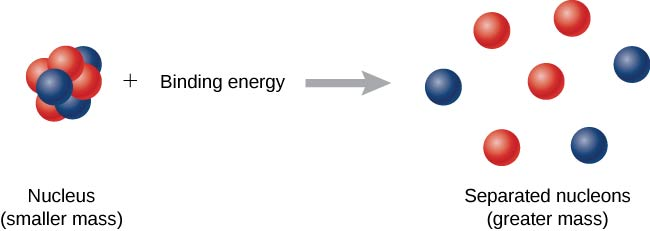Mass Defect and Binding Energy of the Deuteron Calculate the mass defect and the binding energy of the deuteron. The mass of the deuteron is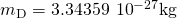or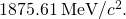Solution From (Figure), the mass defect for the deuteron is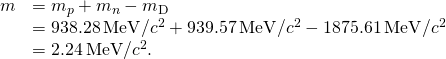The binding energy of the deuteron is then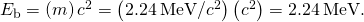Over two million electron volts are needed to break apart a deuteron into a proton and a neutron. This very large value indicates the great strength of the nuclear force. By comparison, the greatest amount of energy required to liberate an electron bound to a hydrogen atom by an attractive Coulomb force (an electromagnetic force) is about 10 eV.

### Graph of Binding Energy per Nucleon

In nuclear physics, one of the most important experimental quantities is the binding energy per nucleon (BEN), which is defined by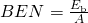This quantity is the average energy required to remove an individual nucleon from a nucleus—analogous to the ionization energy of an electron in an atom. If the BEN is relatively large, the nucleus is relatively stable. BEN values are estimated from nuclear scattering experiments.

A graph of binding energy per nucleon versus atomic number A is given in (Figure). This graph is considered by many physicists to be one of the most important graphs in physics. Two notes are in order. First, typical BEN values range from 6–10 MeV, with an average value of about 8 MeV. In other words, it takes several million electron volts to pry a nucleon from a typical nucleus, as compared to just 13.6 eV to ionize an electron in the ground state of hydrogen. This is why nuclear force is referred to as the “strong” nuclear force.

Second, the graph rises at low A, peaks very near iron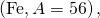and then tapers off at high A. The peak value suggests that the iron nucleus is the most stable nucleus in nature (it is also why nuclear fusion in the cores of stars ends with Fe). The reason the graph rises and tapers off has to do with competing forces in the nucleus. At low values of A, attractive nuclear forces between nucleons dominate over repulsive electrostatic forces between protons. But at high values of A, repulsive electrostatic forces between forces begin to dominate, and these forces tend to break apart the nucleus rather than hold it together.

In this graph of binding energy per nucleon for stable nuclei, the BEN is greatest for nuclei with a mass near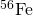. Therefore, fusion of nuclei with mass numbers much less than that of Fe, and fission of nuclei with mass numbers greater than that of Fe, are exothermic processes.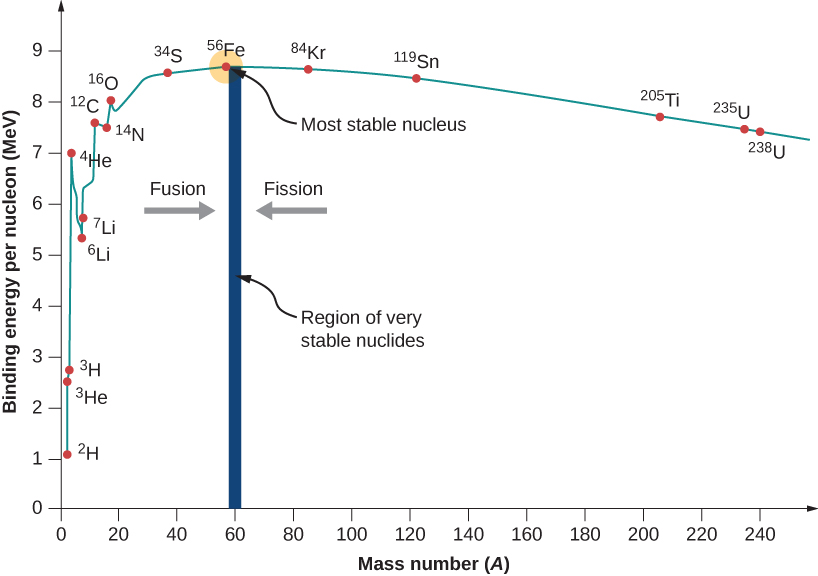As we will see, the BEN-versus-A graph implies that nuclei divided or combined release an enormous amount of energy. This is the basis for a wide range of phenomena, from the production of electricity at a nuclear power plant to sunlight.

Tightly Bound Alpha Nuclides Calculate the binding energy per nucleon of an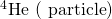.

Strategy Determine the total binding energy (BE) using the equation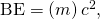whereis the mass defect. The binding energy per nucleon (BEN) is BE divided by A.

Solution For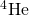, we have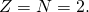The total binding energy is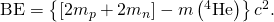These masses are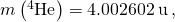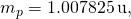and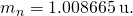Thus we have,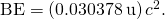Noting that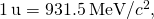we find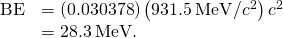Since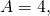the total binding energy per nucleon is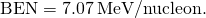Significance Notice that the binding energy per nucleon foris much greater than for the hydrogen isotopes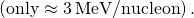Therefore, helium nuclei cannot break down hydrogen isotopes without energy being put into the system.

Check Your Understanding If the binding energy per nucleon is large, does this make it harder or easier to strip off a nucleon from a nucleus?

harder

### Summary

• The mass defect of a nucleus is the difference between the total mass of a nucleus and the sum of the masses of all its constituent nucleons.
• The binding energy (BE) of a nucleus is equal to the amount of energy released in forming the nucleus, or the mass defect multiplied by the speed of light squared.
• A graph of binding energy per nucleon (BEN) versus atomic number A implies that nuclei divided or combined release an enormous amount of energy.
• The binding energy of a nucleon in a nucleus is analogous to the ionization energy of an electron in an atom.

### Conceptual Questions

Explain why a bound system should have less mass than its components. Why is this not observed traditionally, say, for a building made of bricks?

A bound system should have less mass than its components because of energy-mass equivalence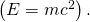If the energy of a system is reduced, the total mass of the system is reduced. If two bricks are placed next to one another, the attraction between them is purely gravitational, assuming the bricks are electrically neutral. The gravitational force between the bricks is relatively small (compared to the strong nuclear force), so the mass defect is much too small to be observed. If the bricks are glued together with cement, the mass defect is likewise small because the electrical interactions between the electrons involved in the bonding are still relatively small.

Why is the number of neutrons greater than the number of protons in stable nuclei that have an A greater than about 40? Why is this effect more pronounced for the heaviest nuclei?

To obtain the most precise value of the binding energy per nucleon, it is important to take into account forces between nucleons at the surface of the nucleus. Will surface effects increase or decrease estimates of BEN?

Nucleons at the surface of a nucleus interact with fewer nucleons. This reduces the binding energy per nucleon, which is based on an average over all the nucleons in the nucleus.

### Problems

How much energy would be released if six hydrogen atoms and six neutrons were combined to form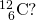92.4 MeV

Find the mass defect and the binding energy for the helium-4 nucleus.is among the most tightly bound of all nuclides. It makes up more than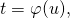of natural iron. Note thathas even numbers of protons and neutrons. Calculate the binding energy per nucleon forand compare it with the approximate value obtained from the graph in (Figure).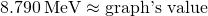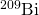is the heaviest stable nuclide, and its BEN is low compared with medium-mass nuclides. Calculate BEN for this nucleus and compare it with the approximate value obtained from the graph in (Figure).

(a) Calculate BEN for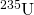, the rarer of the two most common uranium isotopes; (b) Calculate BEN for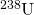. (Most of uranium is.)

a. 7.570 MeV; b.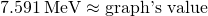The fact that BEN peaks at roughly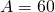implies that the range of the strong nuclear force is about the diameter of this nucleus.

(a) Calculate the diameter ofnucleus.

(b) Compare BEN for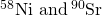. The first is one of the most tightly bound nuclides, whereas the second is larger and less tightly bound.

### Glossary

binding energy (BE)
energy needed to break a nucleus into its constituent protons and neutrons
binding energy per nucleon (BEN)
energy need to remove a nucleon from a nucleus
mass defect
difference between the mass of a nucleus and the total mass of its constituent nucleons